Next: Molecular Quantum Mechanics Up: Some Analytically Soluble Problems Previous: The Rigid Rotor

## The Hydrogen Atom

Finally, consider the hydrogen atom as a proton fixed at the origin, orbited by an electron of reduced mass. The potential due to electrostatic attraction is
(126)

in SI units. The kinetic energy term in the Hamiltonian is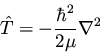(127)

so we write out the Schrödinger equation in spherical polar coordinates as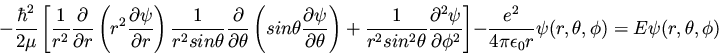(128)

It happens that we can factor
into
, where
are again the spherical harmonics. The radial part R(r) then can be shown to obey the equation(129)

which is called the radial equation for the hydrogen atom. Its (messy) solutions are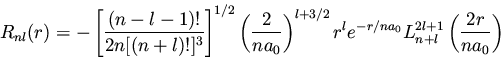(130)

where
, and a0 is the Bohr radius,
. The functions Ln+l2l+1(2r/na0) are the associated Laguerre functions. The hydrogen atom eigenvalues are
(131)

There are relatively few other interesting problems that can be solved analytically. For molecular systems, one must resort to approximate solutions.Next: Molecular Quantum Mechanics Up: Some Analytically Soluble Problems Previous: The Rigid Rotor